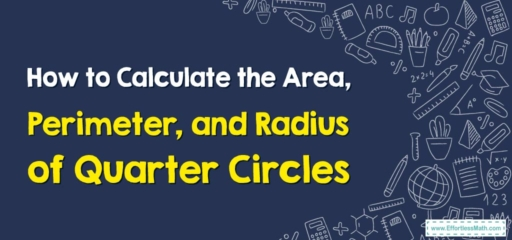# How to Calculate the Area, Perimeter, and Radius of Quarter Circles

Today, we're focusing on quarter circles, a particular type of sector of a circle. We'll learn how to calculate their area and perimeter, and even reverse-engineer the radius if we know these properties!## 1. Understanding Quarter Circles

A quarter circle is a sector of a circle that represents one-fourth of the circle. Because it’s a quarter of a circle, the central angle that generates a quarter circle is $$90$$ degrees.

## 2. Calculating the Area, Perimeter, and Radius

The area and perimeter (also called the circumference in full circles) are fractions of the respective measures of a full circle. The radius of a quarter circle is the same as that of the full circle it is a part of.

## Step-By-Step Guide to Calculating the Area, Perimeter, and Radius of Quarter Circles

Let’s break down the process:

### Step 1: Calculate the Area

The area of a quarter circle with radius r is given by: Area $$= 0.25\times π\times r^2$$.

### Step 2: Calculate the Perimeter

The perimeter of a quarter circle is a bit tricky. It includes the arc length (one-fourth of the circumference of the whole circle) and twice the radius (the two straight edges). So, the formula is: Perimeter $$= 0.5\times π\times r + 2r$$.

### Step 3: Find the Radius

If you know the area $$(A)$$ or perimeter $$(P)$$ of the quarter circle, you can rearrange the formulas to solve for the radius:

• From the area: $$r = \sqrt(\frac{(4A)}{(π)})$$
• From the perimeter: $$r = P / (2 + 0.5π)$$

For example, let’s say you have a quarter circle with radius 4 units:

1. Find the area: Area $$= 0.25\times π\times 4^2 = 4π$$ square units.
2. Find the perimeter: Perimeter $$= 0.5\times π\times 4 + 2\times 4 = 2π + 8$$ units.

As always, keep practicing, keep exploring, and enjoy your mathematical journey!

In this blog post, we’ve explained how to calculate the area, perimeter, and radius of quarter circles. This practical geometric skill can help you with a variety of math problems and real-world applications. Keep practicing, and you’ll master this in no time. Happy calculating!

### What people say about "How to Calculate the Area, Perimeter, and Radius of Quarter Circles - Effortless Math: We Help Students Learn to LOVE Mathematics"?

No one replied yet.

X
30% OFF

Limited time only!

Save Over 30%

SAVE $5 It was$16.99 now it is \$11.99